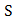# A force–time graph for a linear motion is shown in figure where the segments are circular. The linear momentum gained between zero andisa)b)c)d)## Question ID - 52067 :- A force–time graph for a linear motion is shown in figure where the segments are circular. The linear momentum gained between zero andisa)b)c)d)3537

 (b) As the area above the time axis is numerically equal to area below the time axis therefore net momentum gained by body will be zero because momentum is a vector quantity

Next Question :

 A particle is released from a height. At certain height its kinetic energy is three times its potential energy. The height and speed of the particle at that instant are respectively a)b)c)d)